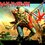# Euler's proof of the infinitude of primes

Almost all of us are familiar with Euclid's proof regarding the infinitude of primes but in this post I will discuss the way Leonhard Euler approached it.I didn't find this proof on anywhere on Brilliant so I decided to do a post on this.Leonhard Euler Image Credit:Wikipedia

$\textbf{Proof:}$According to the fundamental theorem of Arithmetic every integer$n$ can be represented as the product of prime numbers $n = p_1^{a_1} p_2^{a_2}...... p_k{a_k}$.

Let us assume there are finitely many primes $p_1.

Let us denote by $N$ the following product:

$N = (1 + \frac{1}{p_1}+\frac{1}{p_1^{2}}+\frac{1}{p_1^{3}}+...)(1 + \frac{1}{p_2}+\frac{1}{p_2^{2}}+\frac{1}{p_2^{3}}+..)....$ $....(1 + \frac{1}{p_n}+\frac{1}{p_n^{2}}+\frac{1}{p_n^{3}}+...)$

Now for each term in the product we can evaluate the sum of the expression using infinite GP(since $\frac{1}{p_i}<1$ ) to be : $(1 + \frac{1}{p_i}+\frac{1}{p_i^{2}}+\frac{1}{p_i^{3}}+...) = \frac{p_i}{p_i-1}$

So clearly the expression on the right hand side is finite...so N corresponds to a finite expression. - (1)

But now if we multiply out the terms in n then we will get the sum of the reciprocals of all the natural numbers because every natural number is the product of primes and each distinct combination of primes lead to a natural number.Clearly we can see that the product of the expressions in N leads to the occurrence of every possible combination of the prime numbers in the denominator and hence the reciprocals of all natural numbers . That is

$N = (1 + \frac{1}{p_1}+\frac{1}{p_1^{2}}+\frac{1}{p_1^{3}}+...)(1 + \frac{1}{p_2}+\frac{1}{p_2^{2}}+\frac{1}{p_2^{3}}+..)....$ $....(1 + \frac{1}{p_n}+\frac{1}{p_n^{2}}+\frac{1}{p_n^{3}}+...)$

$N = 1+\frac{1}{2}+\frac{1}{3}+........$

We know that this series is diverging which contracts equation 1 which proved the finiteness of N.Hence we have a contradiction!!

So there are infinitely many primes.

$\textbf{Personal Note:}$It will be extremely helpful for me if Mr.Calvin Lin and other staff members offer a bit of constructive criticism of my posts and offer some advice on how I can improve my writing skills.My peers are also welcome to do the same..Here are my previous posts:

Partitioning of integers

Fermat's Method of infinite descent

Stacking problemsNote by Eddie The Head
7 years, 3 months ago

This discussion board is a place to discuss our Daily Challenges and the math and science related to those challenges. Explanations are more than just a solution — they should explain the steps and thinking strategies that you used to obtain the solution. Comments should further the discussion of math and science.

When posting on Brilliant:

• Use the emojis to react to an explanation, whether you're congratulating a job well done , or just really confused .
• Ask specific questions about the challenge or the steps in somebody's explanation. Well-posed questions can add a lot to the discussion, but posting "I don't understand!" doesn't help anyone.
• Try to contribute something new to the discussion, whether it is an extension, generalization or other idea related to the challenge.
• Stay on topic — we're all here to learn more about math and science, not to hear about your favorite get-rich-quick scheme or current world events.

MarkdownAppears as
*italics* or _italics_ italics
**bold** or __bold__ bold
- bulleted- list
• bulleted
• list
1. numbered2. list
1. numbered
2. list
Note: you must add a full line of space before and after lists for them to show up correctly
paragraph 1paragraph 2

paragraph 1

paragraph 2

[example link](https://brilliant.org)example link
> This is a quote
This is a quote
    # I indented these lines
# 4 spaces, and now they show
# up as a code block.

print "hello world"
# I indented these lines
# 4 spaces, and now they show
# up as a code block.

print "hello world"
MathAppears as
Remember to wrap math in $$ ... $$ or $ ... $ to ensure proper formatting.
2 \times 3 $2 \times 3$
2^{34} $2^{34}$
a_{i-1} $a_{i-1}$
\frac{2}{3} $\frac{2}{3}$
\sqrt{2} $\sqrt{2}$
\sum_{i=1}^3 $\sum_{i=1}^3$
\sin \theta $\sin \theta$
\boxed{123} $\boxed{123}$

## Comments

Sort by:

Top Newest

I like User Submitted posts on Brilliant...I get to learn so many new things! If I may make a suggestion, could you write about methods of finding extremes of a function as one of your next posts? I was wondering what methods exist and had put up a post for the community, but didn't get any satisfactory responses. See https://brilliant.org/discussions/thread/extremes-of-a-function/?ref_id=105581

- 7 years, 3 months ago

Log in to reply

Do you mean finding the maxima and minima using calculus? That's a great idea... actually I was thinking about doing one on Newton' method of approximating roots of a function...

- 7 years, 3 months ago

Log in to reply

I meant the various ways by which we can find the extremes, because sometimes, calculus is overkill for a question, but some inequality, say Cauchy Schwarz, makes short work of it. So I wanted a sort of guideline as to which technique to choose when.

- 7 years, 3 months ago

Log in to reply

Maybe we can start a new thread for that where many people will be able to express their approach towards these problems???That would be cool..

- 7 years, 3 months ago

Log in to reply

Yea, that's what I had in mind...but my post didn't garner many comments or any real discussion...I need more re shares.

- 7 years, 3 months ago

Log in to reply

×

Problem Loading...

Note Loading...

Set Loading...